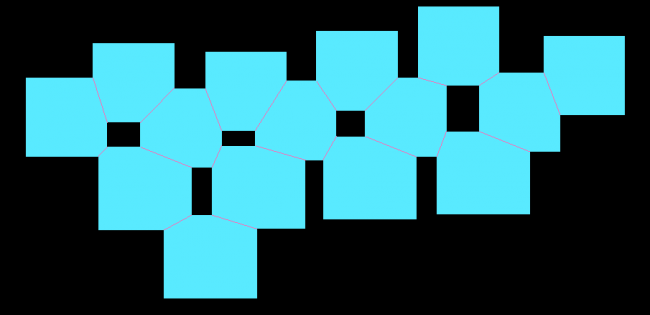#### Howdy, Stranger!

We are about to switch to a new forum software. Until then we have removed the registration on this forum.

# Intersected polygons diagonals

edited March 2016

Hello,

I am working with intersections of curves. I tried toxiclibs and hemesh libraries at first. But after lots of errors I tried to write a simple script using python in grasshopper. As a result, I noticed that there were issues with data tree, that I could not solve in processing or simple because of my skills of programming.

Long story short, I have an array of polygons. When they intersect, their corners rotate 90 degrees downwards.

Simple, but extensive to write a script in processing. For now I have quite fast script in grasshopper. The main component calculates intersection between curves ant returns diagonals in data tree. When I tried to play with movement and physics, I saw that I need to realize my ideas in processing. As a result, I want to rewrite the script in processing...

Here are diagonals (in purple color) I am working on:The intersection function for getting intersection diagonals was written in python:

``````import ghpythonlib.components as ghcomp
import rhinoscriptsyntax as rs

def ctr(crv):
pts = ghcomp.Explode(crv) //polygons are exploded into segmens
pts = ghcomp.CullDuplicates(pts,0.001) //duplicate lines are deleted
return ghcomp.Average(pts)

pts = []
lines = []

ctr_c1 = ctr(C1)

for crv in C2:
if ctr(crv) != ctr_c1:
int = ghcomp.CurveXCurve(C1, crv) //intersection of curves is calculated
if int:
[pts.append(x) for x in int]
``````

The script returns diagonals of intersected curves in a data tree. By data tree I mean that there are 15 polygons in the picture. And the script returns the output saying that 1st curve has 2 objects as lines, 2nd curve has 3 objects as lines, 3rd curve has 1 object as line and so on. Each polygon is associated with intersection diagonals.

Could you help me to rewrite this python script in processing? If not, maybe you have references for similar scripts in processing using any relevant library?

• ``````import ghpythonlib.components as ghcomp
import rhinoscriptsyntax as rs
``````

So do you happen to have those libraries available in Java too?! #-o

• This is the case, that I am questioning if anybody has experience with libraries that could help solving intersection issues.

• edited February 2014

Are these polygons all rectangles? An is the diagonal you talk about, the constructed line between the 2 outer connected points, while the 2 inner overlapping points are discarded? It would also help if you provided some concise runnable code that produced the polygons.

• This function will calculate the intersection points between two rectangles. The link will be your 'diagonal'

``````/**
* If two boxes overlap then the overlap region is another box. This method is used to
* calculate the coordinates of the overlap. <br>
*
* [ax0, ay0]/[ax1, ay1] top-left and bottom-right corners of rectangle A
* [bx0, by0]/[bx1, by1] top-left and bottom-right corners of rectangle B
*
* If the returned array has a length:
* 0 then they do not overlap <br>
* 4 then these are the coordinates of the top-left and bottom-right corners of the overlap region.
*
*/
public float[] box_box_p(float ax0, float ay0, float ax1, float ay1, float bx0, float by0, float bx1, float by1){
float[] result = null;
float topA = min(ay0, ay1);
float botA = max(ay0, ay1);
float leftA = min(ax0, ax1);
float rightA = max(ax0, ax1);
float topB = min(by0, by1);
float botB = max(by0, by1);
float leftB = min(bx0, bx1);
float rightB = max(bx0, bx1);

if(botA <= topB  || botB <= topA || rightA <= leftB || rightB <= leftA)
return new float;

float leftO = (leftA < leftB) ? leftB : leftA;
float rightO = (rightA > rightB) ? rightB : rightA;
float botO = (botA > botB) ? botB : botA;
float topO = (topA < topB) ? topB : topA;
return new float[] {leftO, topO, rightO, botO};
}
``````